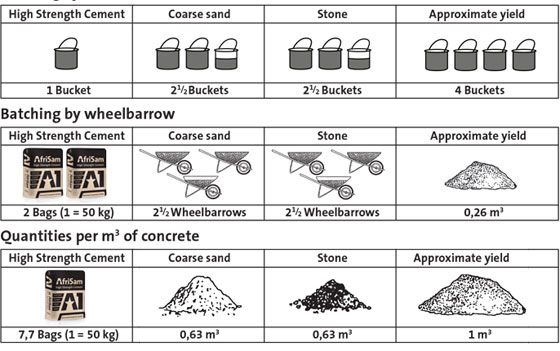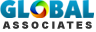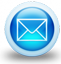# Usefulness of construction formulas and equations for building projects

## Home » Construction take-off sheets » Earthwork Volume Calculation Sheet

The formulas & equations are essential for construction professionals to work out the quantities as well as cost & subtotals of building material. These formulas can be used in a wide array of construction projects and design applications which range from reinforced concrete, bridge construction, highway design etc.

A formula refers to equation demonstrating one variable as an amalgamation of other variable(s) with the use of algebraic operations like add, subtract, multiply, divide, raise to a power, apply the natural logarithm as well as the cosine, or other mixture of operations.

Various issues related to construction can be easily resolved with proper application of these formulas.

The insight of the construction industry will be enhanced with professionalism and performance through effective use of formulas.

Concrete: Width x Length x Height, divided by 27 = number of yards of concrete needed

Foundation Masonry Block: 8" high / 16" long / 3/8" mortar normal joint height.

Roofing: Width x length, divided by 100 = number of squares of shingles needed.

Siding: Width x Height, divided by 100 Square Feet = number of squares of siding needed.

Carpet: Width of room x length of room, divided by 9 = number of square feet needed for room.

Wood Siding: Width of board minus distance of lapping = coverage of wood siding per board.

Width of area to be sided x height of area to be sided, divided by coverage calculated above = lineal feet of siding you need.

Brick: 7 bricks = one square feet of coverage.

Width of area to be covered x height of area to be covered, divided by 7 = number of bricks needed.

Elevation Conversion: Elevation measured in 10ths per foot. 1 of elevation = one foot or 12" equals one foot.

Normal measurement 12 inches per foot.

Convert Elevation to Inches: One Foot of Elevation measurement equals 1.2 inches of normal measurements.

Common Terms Used: Board feet = (thickness (in inches) x width (in inches) x length (in inches)) divided by 12 Lineal feet = length only of board.Image Courtesy: afrisam.co.za

# Takeoff Tools

DISCLAIMER: "Autodesk, AutoCAD, Revit, Navisworks, Ecotect, QTO, DWG, 3ds Max, are registered trademarks or trademarks of Autodesk, Inc. All other brand names, product names, or trademarks belong to their respective holders. © 2010 Autodesk, Inc. All rights reserved.

Site Design & Develop by